Monday, September 27, 2021
Home > geometry > SSC CGL Geometry Lines And Angles Set 1

# SSC CGL Geometry Lines And Angles Set 1SSC CGL Geometry Lines And Angles Set 1 : Ques No 1

Find the value of θ in the figure below.Options:

A. 30 degrees

B. 45 degrees

C. 60 degrees

D. 15 degrees

Solution: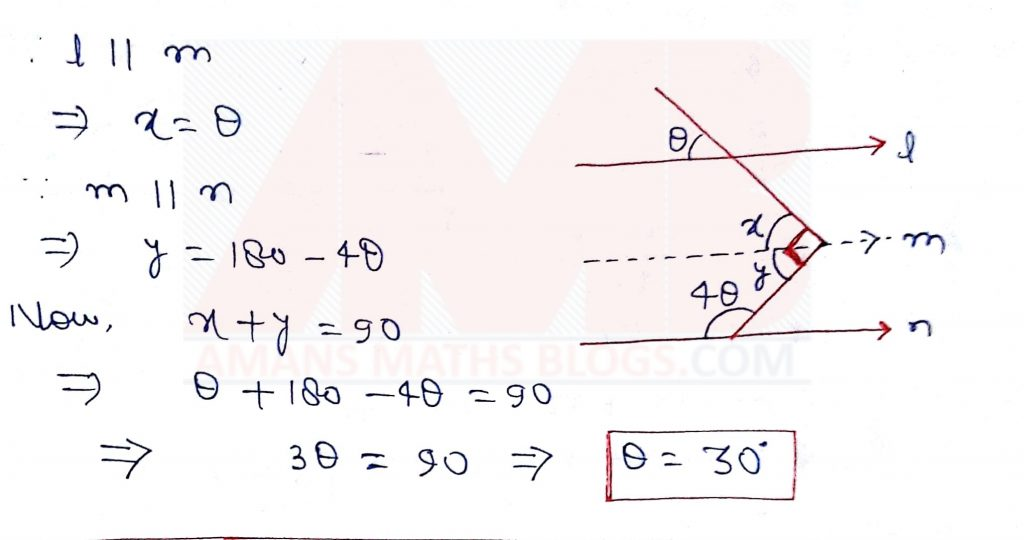SSC CGL Geometry Lines And Angles Set 1 : Ques No 2

Find the value of θ in the figure below.Options:

A. 10 degrees

B. 25 degrees

C. 30 degrees

D. 45 degrees

Solution: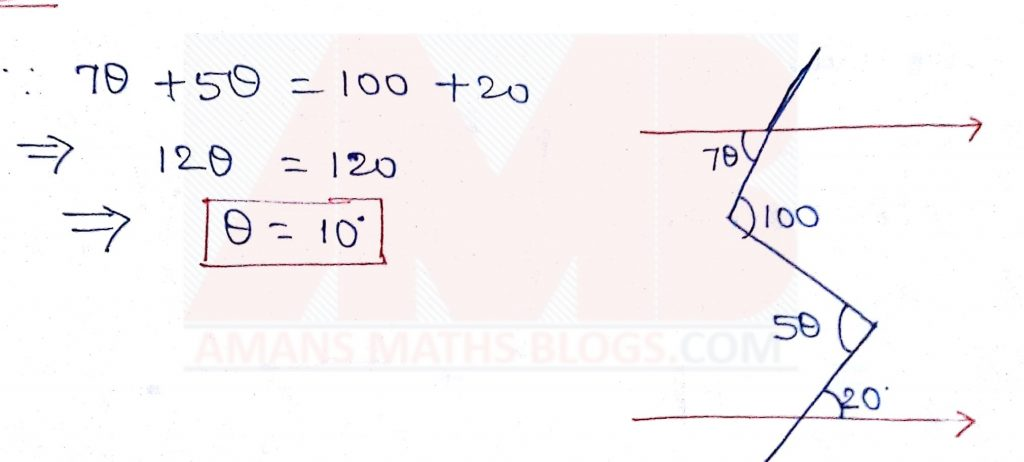SSC CGL Geometry Lines And Angles Set 1 : Ques No 3

Find the value of θ in the figure below.Options:

A. 30 degrees

B. 45 degrees

C. 60 degrees

D. 15 degrees

Solution:SSC CGL Geometry Lines And Angles Set 1 : Ques No 4

Find the value of θ in the figure below.Options:

A. 30 degrees

B. 60 degrees

C. 90 degrees

D. 15 degrees

Solution:SSC CGL Geometry Lines And Angles Set 1 : Ques No 5

Find the value of θ in the figure below.Options:

A. 30 degrees

B. 25 degrees

C. 40 degrees

D. 35 degrees

Solution:SSC CGL Geometry Lines And Angles Set 1 : Ques No 6

Find the value of (x y) in the figure below.Options:

A. 300 degrees

B. 450 degrees

C. 260 degrees

D. 150 degrees

Solution:SSC CGL Geometry Lines And Angles Set 1 : Ques No 7

If α+β = 170°, then find the value of x in the figure below.Options:

A. 130 degrees

B. 135 degrees

C. 120 degrees

D. 150 degrees

Solution: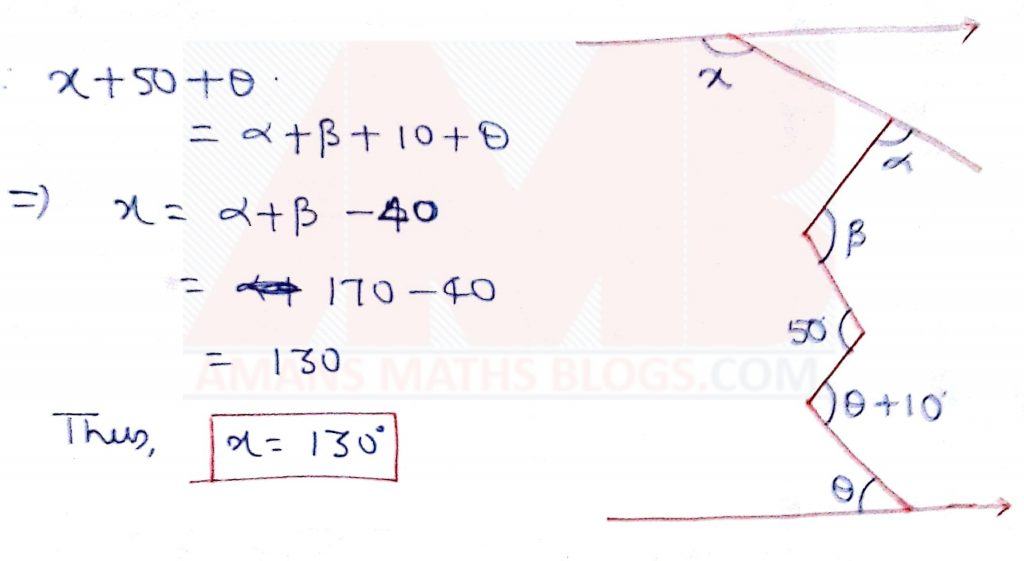SSC CGL Geometry Lines And Angles Set 1 : Ques No 8

Find the value of x in the figure below.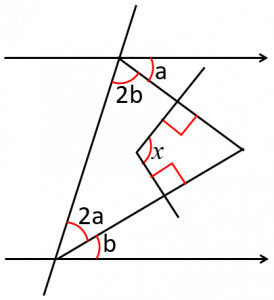Options:

A. 130 degrees

B. 140 degrees

C. 120 degrees

D. 150 degrees

Solution:SSC CGL Geometry Lines And Angles Set 1 : Ques No 9

Find the value of x in the figure below.Options:

A. 110 degrees

B. 120 degrees

C. 130 degrees

D. 140 degrees

Solution:SSC CGL Geometry Lines And Angles Set 1 : Ques No 10

Find the value of x in the figure below.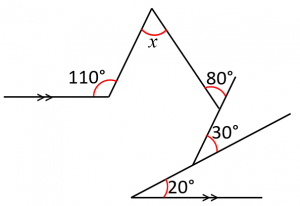Options:

A. 30 degrees

B. 45 degrees

C. 60 degrees

D. 15 degrees

Solution: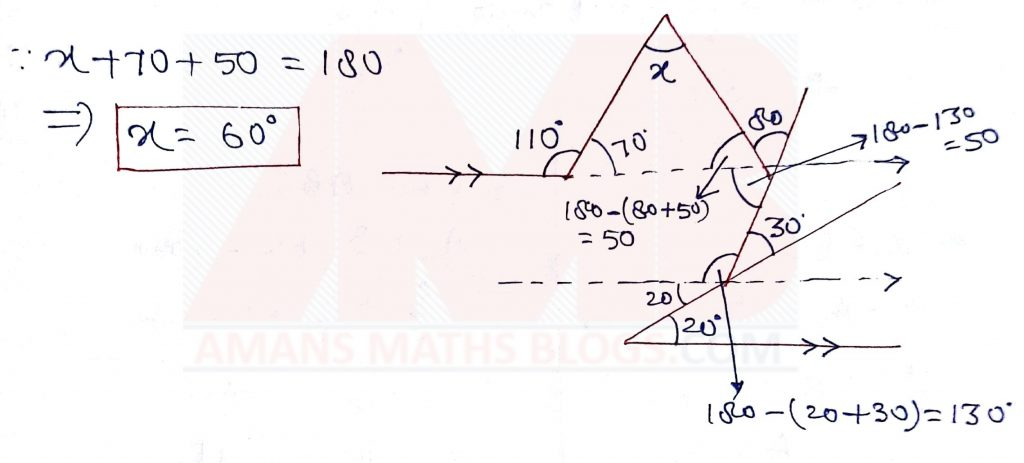SSC CGL Geometry Lines And Angles Set 1 : Ques No 11

The complement angle of 27o20′ is

Options:

A. 63o20′

B. 63o40′

C. 62o40′

D. 62o20′

SSC CGL Geometry Lines And Angles Set 1 : Ques No 12

An angle is 46o less than its complement, the angle is

Options:

A. 46o

B. 68o

C. 56o

D. 22o

SSC CGL Geometry Lines And Angles Set 1 : Ques No 13

If two supplementary angles are in the ratio 7 : 8, then the difference of the angles is

Options:

A. 12o

B. 36o

C. 84o

D. 96o

SSC CGL Geometry Lines And Angles Set 1 : Ques No 14

If the two angles (x – 2)o and (2x + 5)o are supplementary angles, then the complementary angle of (x + 3)is

Options:

A. 57o

B. 23o

C. 60o

D. 30o

SSC CGL Geometry Lines And Angles Set 1 : Ques No 15

If the two angles (2x – 7)o and (x + 4)o are complementary angles, then the supplementary angle of (x – 1)is

Options:

A. 130o

B. 150o

C. 160o

D. 120o

SSC CGL Geometry Lines And Angles Set 1 : Ques No 16

If the difference between two complementary angles is 180o, then the supplementary angle of larger angle is

Options:

A. 120o

B. 122o

C. 126o

D. 128o